# What is Bar Bending Schedule | BBS Step by Step Preparation | BBS formulas | BBS Sample Excel Sheet

In this Article today we will talk about the What is Bar Bending Schedule | BBS Step By Step Preparation | BBS Formulas | Reinforcement Calculation Excel | BBS Sample Excel Sheet.

## What is Bar Bending Schedule (BBS) ?

Bar Bending Schedule is a detailed list of bent reinforcement bars given in any structural concrete element. Bar Bending Schedule Contains bar mark, Diameter, length, shape, and weight. The Bar bending is a process of cutting and bending reinforcement steel bar into Desire shape as per structural drawing was given by structural engineer for various structural elements like footing, column, beam, slab, etc.

A Bar Bending Schedule helps site engineer to estimate required quantities of the various diameter of bars used in construction. It helps the engineer to check the reinforcement work done by the contractor.

## Advantages of Bar Bending Schedule in Construction:

1. Provides better estimation of reinforcement used in each structural concrete member. You can also calculate the overall reinforcement required for the construction project.
2. It helps engineer in the procurement of reinforcement steel and better stock management. BBS Formulas
3. Bar Bending Schedule Provides exact estimated quantity required. So, at a construction site, wastage can be controlled by careful supervising of the contractor with the help of Bar Bending Schedule. Reinforcement Calculation Excel
4. Bar Bending Schedule makes cutting, bending of reinforcement easy at a factory and can be directly transported to the Construction site. This will not only reduce the wastage of reinforcement steel but also help us reduce labor cost. Though, it helping in reducing the overall cost of the construction project.
5. This helps site engineer or quality inspector to check and approve reinforcement work with better quality control. BBS Step By Step Preparation
6. Billing can be done really fast and easy using Bar Bending Schedule. It also makes easy for clients to approve bills for Payment.

## Step by Step Preparation of Bar Bending Schedule:

### Step 1: Identification of Members (Steel Bars)

Each different type of Steel bar or Member is usually tagged with a number or bar mark in the structural drawing. Now, open a structural drawing and list down all the shapes of bar you see in the structural drawing. Now mark the diameter of Member in the table.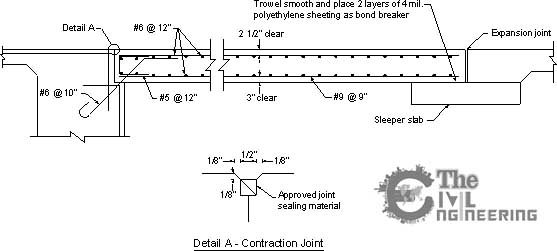The above image shows the number of bars like #5, #6, #9, etc, along with Día of the Bar (e.g. #[email protected]”). BBS Step By Step Preparation

### Step 2: Count the Number of bars

Now, Count the number of each different shapes of the bar and note down in bar bending schedule

To calculate a number of bars in big slab structure, you need to check the specific spacing between bars (center to center or c/c, its show in above fig) and the length of the concrete slab in which the bars are distributed.

Number of Bars = (Concrete slab length in which bar is Distributed – 2 X Cover) / (c/c spacing between bars) + 1

For Example:

Suppose, 10 MM bars are distributed in the 10-meter-long slab at 150 mm c/c spacing, Considering the side cover of 50 mm.

Then calculate the number of bars

Number of Bars = [(10000 – 2 X 50) / (150)] + 1 = 66 +1 = 67 Bars of 10 mm Día

### Step 3: Calculate the length of Reinforcement bars

The formula, to calculate the length of those bars.

Length of Bar = Length of Concrete member (direction in which bar is placed) – (2 X Cover) + Development length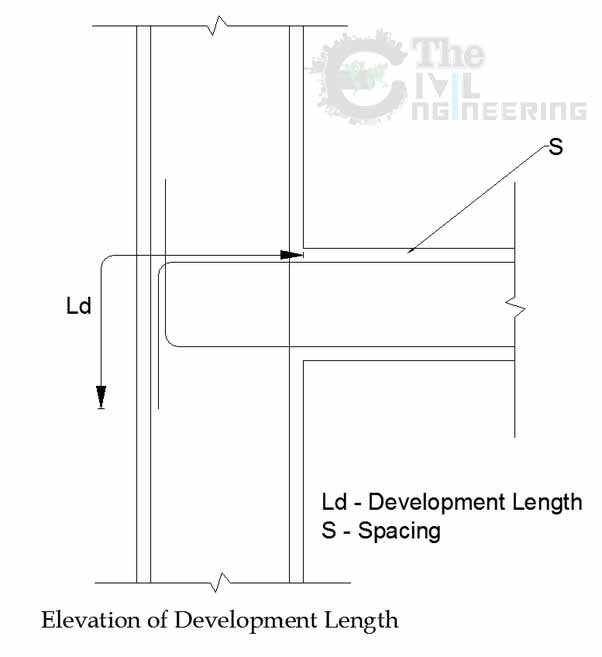#### Development Length Chart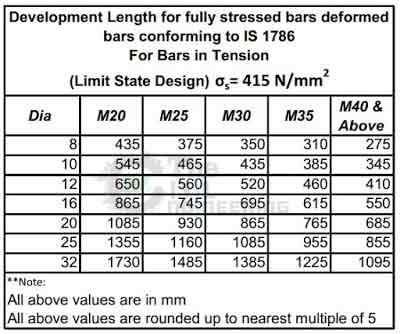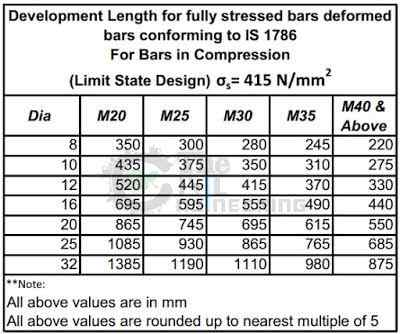### Step 4: Calculate the unit weight of Reinforcement Bar

The formula for Calculating Unit weight of Bar

Unit weight of Bar = Volume of Bar X Density of Bar (7850 kg/m3)

Unit Weight of Reinforcement Bar with Día (D) = D2 / 162

Example, 10 mm Día reinforcement bar Weight = 102 /162 = 0.617 kg/m.

However, you can use the below table to check the Unit weight of different Día Bars. BBS Formulas

### Step 5: Calculate the Weight of particular Shape of Bar

Wight of Reinforcement Bar (Shape of Bar) = No of Bars X Length of Bars X Unit weight of Bar This Formula gives the total Weight of specific shape Bar in Kilogram

## Point to remember for Bar Bending Schedule (Formulas):

### Diameter of Reinforcement Bar (in mm):

The Commonly used diameter of reinforcement bars (in mm):

6mm, 833, 10mm, 12mm, 16mm, 20mm, 25mm, 25mm, 28mm, 32mm, 36mm, 40mm

### Standard Length of Reinforcement Bars (in m)

The Standard length of reinforcement bars is taken 12m

### Weight of Bar Per Length (in Kg/m)

The weight per length of the reinforcement bars is expressed as,

Weight/m = D2/162

For Example: If the diameter of the reinforcement bar is 6mm,

then,

Weight/m = 62 / 162 = 0.22 Kg/m (Note: you should keep the value of D in mm and answer comes in m)

### Weight Per Bar (in Kg per Bar)

The weight per bar can be expressed as,

Weight/bar = Weight of Bar Per m x Standard Length of Bar = 0.22 x 12 = 2.64 Kg/bar. BBS Formulas

### Diameter of Reinforcement Bars (in inches)

#3 Rebar = 3/8 in = 9.525 mm

#4 Rebar = ½ in = 12.70 mm

#5 Rebar = 5/8 in = 15.875 mm

#6 Rebar = ¾ in = 19.05 mm

#7 Rebar = 7/8 in = 22.225 mm

#8 Rebar = 1 in = 25.4 mm

#9 Rebar = 9/8 in = 28.575 mm

### Standard Length of Reinforcement Bar (in feet)

The Standard Length of reinforcement bar is taken as 40 ft

### Weight of Reinforcement Bar Per Length (in Kg/ft)

The weight per bar can be expressed as,

Weight / Length = D2 / 533

For Example:

If the diameter of the reinforcement bar is #4 i-e ½ in or 12.70mm then,

Weight / Length = 12.702 / 533 = 0.302 kg/ft (since, D must be in mm)

Also,

Weight / Length = D2 / 52.896

For Example: If the diameter of the reinforcement bar is #4 then,

Weight / Length = 42/52.896 = 0.302 kg/ft (since D must be in #No.)

### Weight of Reinforcement Bar Per Bar (Kg/Bar)

The weight of reinforcement bar per bar can be expressed as,

Weight/Bar = (D2/533) x Standard Length of Bar

For Example:

If the diameter of the reinforcement bar is 12.70mm (#4) then,

Weight/Bar = (12.702/533) x 40 = 12.10 kg/bar

### Cutting Length of Main Reinforcement Bar with Hook:

Length of Hook = 9d,

where d is the diameter of bar Length of Main Bar = L + 9d + 9d

### Cutting Length of Main Bar with Bend

Length of Bend = 10d – 6d

Length of Main Bar = L + 12d + 12d

### Overlapping

• Neck Column to Footing Overlapping = 40d – 50d
• Column to Column or Beam Overlapping = 50d
• Development Length for Dowel Bars = 16d
• General Overlapping on completion of 12m bar = 50d. BBS Formulas

### Concrete Cover

Cover basically includes a clear cover and effective cover

• Clear cover for footing is taken as 75mm,
• for column 25mm to 50mm,
• for beam 25mm to 50mm
• and for slab 25mm.

### Bend Deduction

• For 45 deg bend = 1d
• For 90 deg Bend = 2d
• For 135 deg Bend = 3d

### Crank Length and Extra Bar Length

• For 45 deg bend , Crank Length = 0.42d
• For 30 deg bend , Crank Length = 0.27d
• For 60 deg bend , Crank Length = 0.58d
• Length of Extra Bar = L/4

## Length of Hook and Total Length of Bar for Different Shapes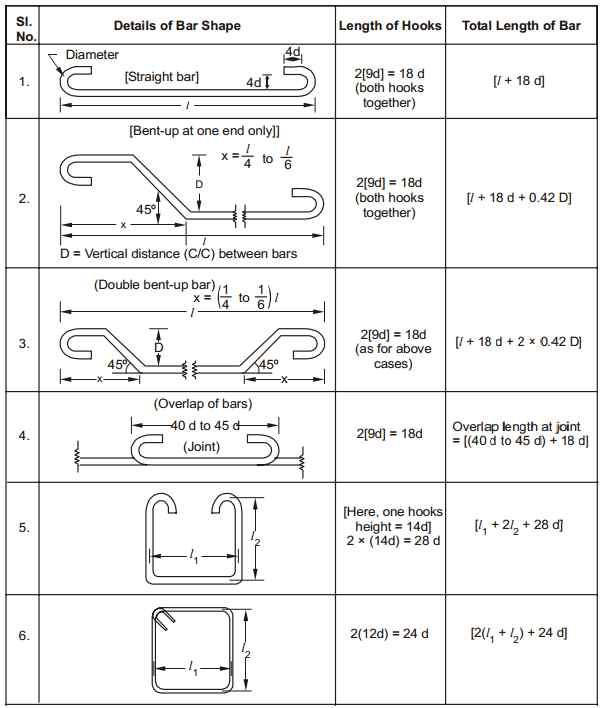## Length of Bent Up Bar for 30, 45 and 60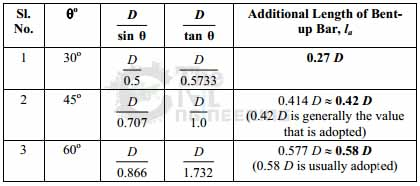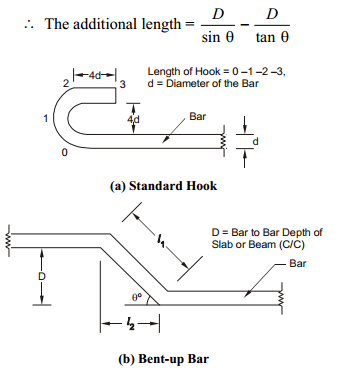## List of Common mistake happens during preparing of Bar Bending Schedule

• Miscounting of the Number of reinforcement Bars. Reinforcement Calculation Excel
• Calculating the length of reinforcement bar incorrectly
• Considering incorrect cover in Beam, Slab, Column, and footings.
• Incorrect counting of bends in reinforcement Bar.

## Tips for Preparing Bar Bending Schedule

• Prepare different Bar Bending Schedule for Different RCC elements (i.e. Divide the table into different parts such as Footing, pedestal, Beam, Column, Slab, etc) to avoid mistakes. Reinforcement Calculation Excel
• Always keep the unit weight of bars Handy. It helps you prepare Bar Bending Schedule.
• Keep thumb rules in mind or handy, that always helps you in cross-checking your Calculation. BBS Sample Excel Sheet
• Such as,
• Approximate quantity of reinforcement steel for small structure varies from 3 to 4 Kg/Sqft of Built-up area. Reinforcement Calculation Excel
• Total Volume of Reinforcement steel for the slab is roughly 1.5% to 2% of the volume of Concrete. (Volume of Steel Reinforcement can be calculated as by dividing the weight by density of steel, that is 7850 Kg/M3)

## The volume of Steel Reinforcement for Different RRC member

For Example, for 10 M3 concrete in Column, considering 2.5% of steel Volume, the column has a 0.25 M3 of steel. Calculate the total weight of steel and steel weight per M3.

Volume of Steel for 10m3 Concrete = 0.25 m3

Wight of Steel Per M3 Concrete = total Volume of steel X Density of Steel (i.e. 7850 Kg/M3)

Weight of steel for 10 M3 concrete = 0.25 X 7850 = 1962.5 Kg.

For 10 m3 Concrete, the total steel required is 1962.5 Kg.

For 1 m3 Concrete, the total Steel required is 196.25 Kg. BBS Sample Excel Sheet

### How to Calculate Rolling Margin of Reinforcement Steel Bar

Cut reinforcement bar into 3 pieces, each length 1 meter Weight Each piece differently i.e. 10 mm Día Bar, 1st one weigh 0.617, 2nd one weighs 0.62, 3rd one weigh 0.625

Now Calculate the rolling margin of Reinforcement bar

## Numerical Example of Bar Bending Schedule:

### Bar Bending Schedule of RCC Beam: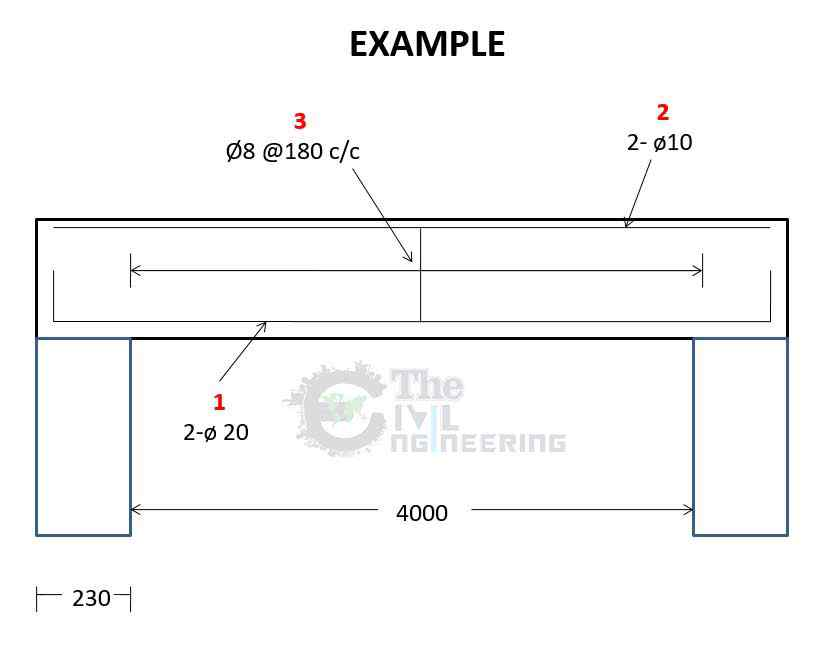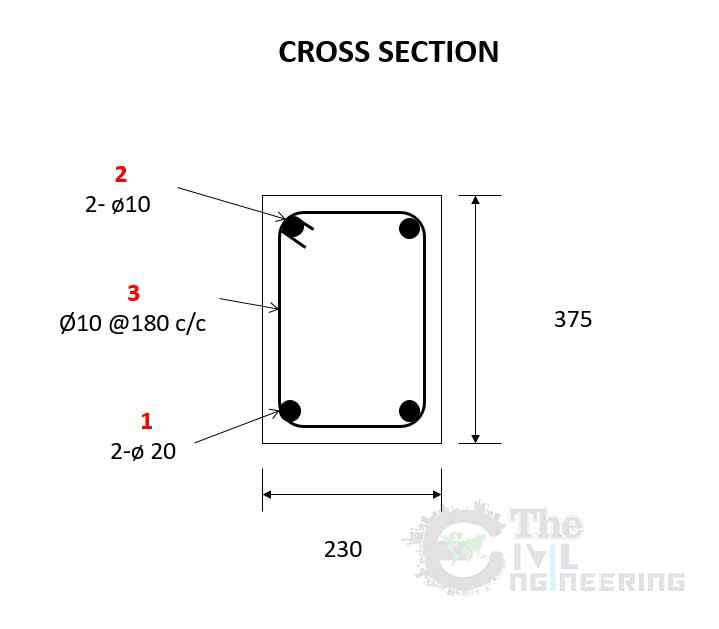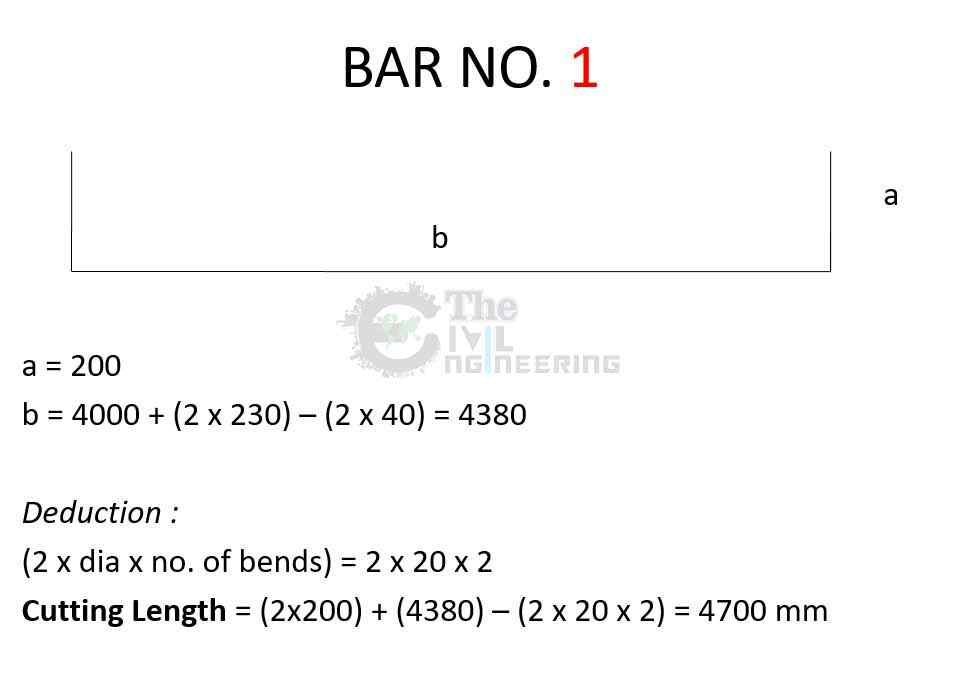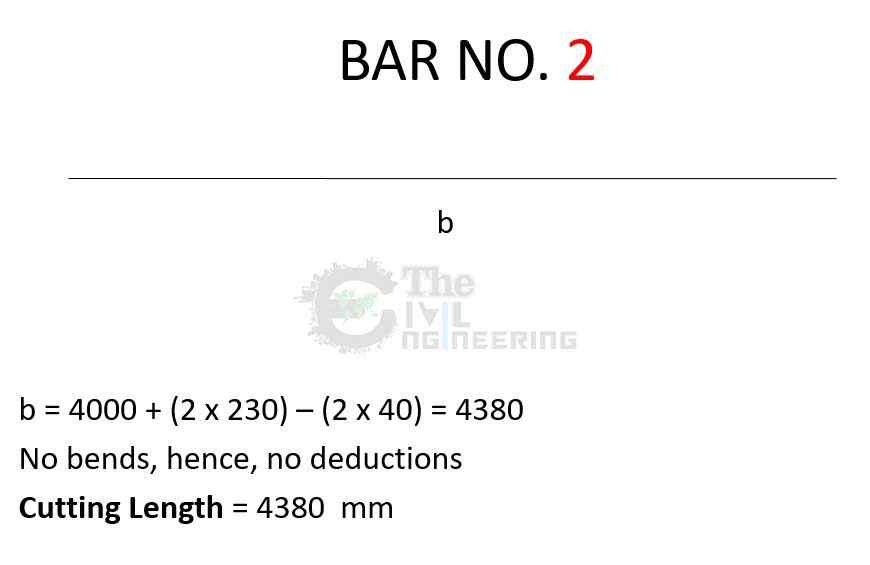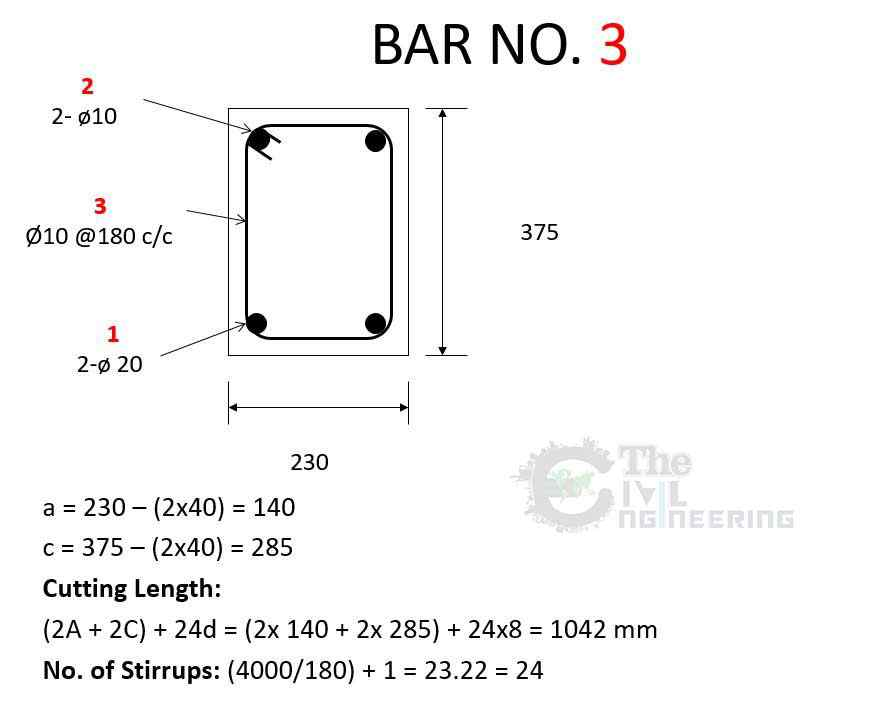## Conclusion:

Full article on What is Bar Bending Schedule | BBS Step By Step Preparation | BBS Formulas | Reinforcement Calculation Excel | BBS Sample Excel Sheet. Thank you for the full reading of this article in “The Civil Engineering” platform in English. If you find this post helpful, then help others by sharing it on social media. If you have any question regarding article please tell me in comments.

1.Hari Ginanjar

Dear Waseem Raja
I am a practically QS for more 20th years from 1992 – 2022. I find these as a complete knowledge of BBS . Thanks for the sharing of knowledge. May Alloh would give you blessings.
Regards.

2.M RAM MOHAN

3.Kelemu demis

Thanks so much

• Thanks for the comments please visit more articles by clicking on “All Posts” tab of the Menu Bar

4.Eng.Bote Tolossa

Very Good , Great Job

• Thanks for the comments please visit more articles by clicking on “All Posts” tab of the Menu Bar

5.Md Akif Ansari

6.Mohammed Tameezul Hussain

Dear wasim
Plz let me know how to prepare bbs with formulas in excel , I.e in each column with formula is to be given so that we can easily get the total weight and cutting length,, I need just format .
Am new to this

• I will try to upload soon regarding excel formulas please keep in touch with us

7.Luckee Yadav

Cant find the BBC Excel sample sheet

• i will upload soon, Thanks for the comments please visit more articles by clicking on “All Posts” tab of the Menu Bar

8.B Madhusudhana Rao

Pl share excel sheet

• Thanks for the comments please visit more articles by clicking on “All Posts” tab of the Menu Bar

9.Akhilesh Bhagat

Provide BBS exel sheet with formula

• I will provide u later, Thanks for the comments please visit more articles by clicking on “All Posts” tab of the Menu Bar